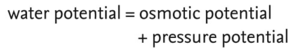# water potential, suction pressure

(redirected from Diffusion Pressure Deficit)
Also found in: Encyclopedia.

## water potential, suction pressure or (formerly) diffusion pressure deficit (DPD)

the tendency of a cell to draw in water from outside by OSMOSIS, the water moving from a higher to a lower water potential. Since pure water at one atmosphere has a water potential of zero, cells drawing in water have a water potential of less than zero. Thus water potential is measured as a negative value, which can be confusing. Readers should be careful when using the terms ‘higher’ or ‘lower’ water potential; ‘more negative’ or ‘less negative’ are more exact statements In mathematical terms water potential is the sum of the OSMOTIC POTENTIAL and the PRESSURE POTENTIAL of a cell:For example, when Osmotic Potential = -9

and Pressure Potential = 4,

Water Potential = - 9 + 4 = - 5.

see OSMOREGULATION.

Site: Follow: Share:
Open / Close